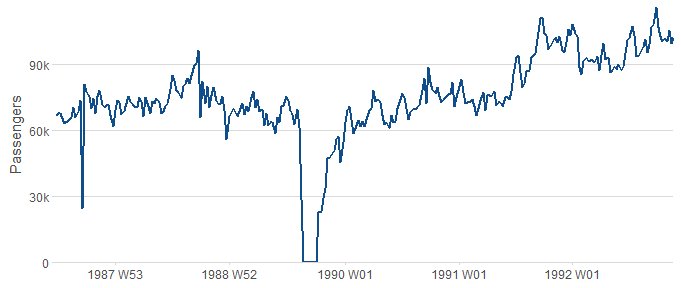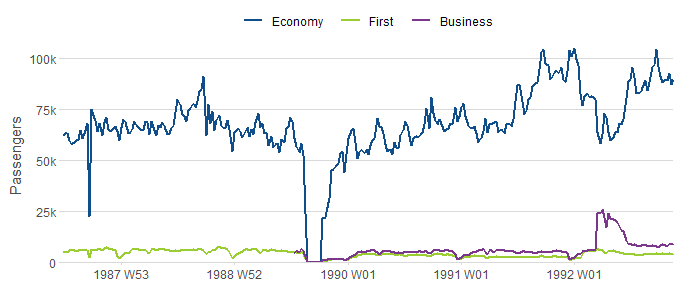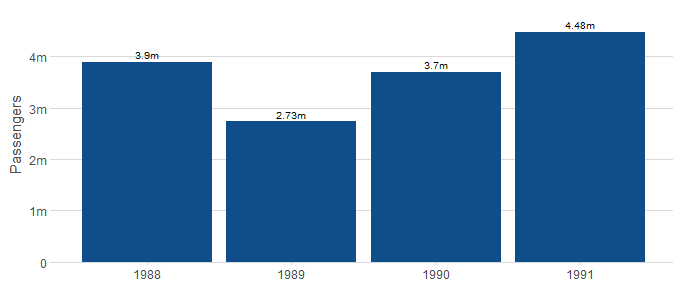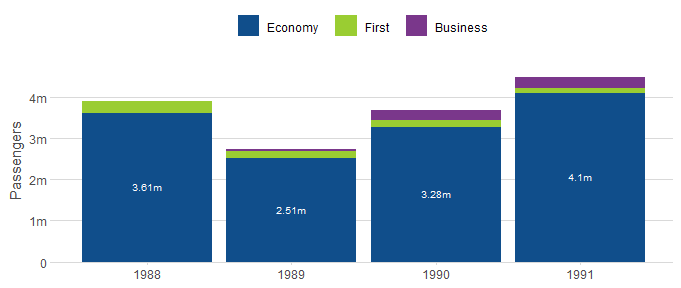# Overview

Use ezplot to quickly create presentation-ready charts that are also useful for exploratory data analysis. By default, ezplot functions aggregate multiple values of y for repeated categories of x, group, facet_y and facet_x.

library(ezplot)
library(tsibble)
#>
#> Attaching package: 'tsibble'
#> The following objects are masked from 'package:base':
#>
#>     intersect, setdiff, union
library(tsibbledata)
suppressPackageStartupMessages(library(dplyr))
suppressPackageStartupMessages(library(lubridate))

## line_plot

line_plot(ansett, x = "Week", y = "Passengers")line_plot(ansett, x = "Week", y = "Passengers", group = "Class")## bar_plot

df = ansett %>%
filter(between(year(Week), 1988, 1991))
bar_plot(df, x = "year(Week)", y = "Passengers")bar_plot(df, x = "year(Week)", y = "Passengers", group = "Class")